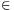Mathematical and Physical Journal
for High Schools
Issued by the MATFUND Foundation
 Already signed up? New to KöMaL?

#Problem A. 463. (October 2008)

A. 463. Let a1<a2<...<an and b1<b2<...<bn be real numbers. Show that(5 pont)

Deadline expired on November 17, 2008.

Solution. Apply induction on n. For n=1 the statement is ea1b1>0 which is obvious. Now suppose n>1 and assume that the statement is true for all smaller values.

Let ci=ai-a1>0. Thenso it is sufficient to prove that the last determinant is positive.

To eliminate the first row, subtract the (n-1)th column from the nth column. Then subtract the (n-2)th column from the (n-1)th column, and so on, finally subtract the first column from the second column. ThenConsider the functionThenBy Lagrange's mean value theorem, there exists a b1<x1<b2 such that f(b2)-f(b1)=(b2-b1)f'(x1), i.e.Repeating the same argument for each column, it can be btained that there exist real numbers xi(bi,bi+1) (1in-1) such thatBy the induction hypothesis, this is positive.

### Statistics:

 4 students sent a solution. 5 points: Nagy 235 János, Nagy 314 Dániel, Tomon István. 1 point: 1 student.

Problems in Mathematics of KöMaL, October 2008